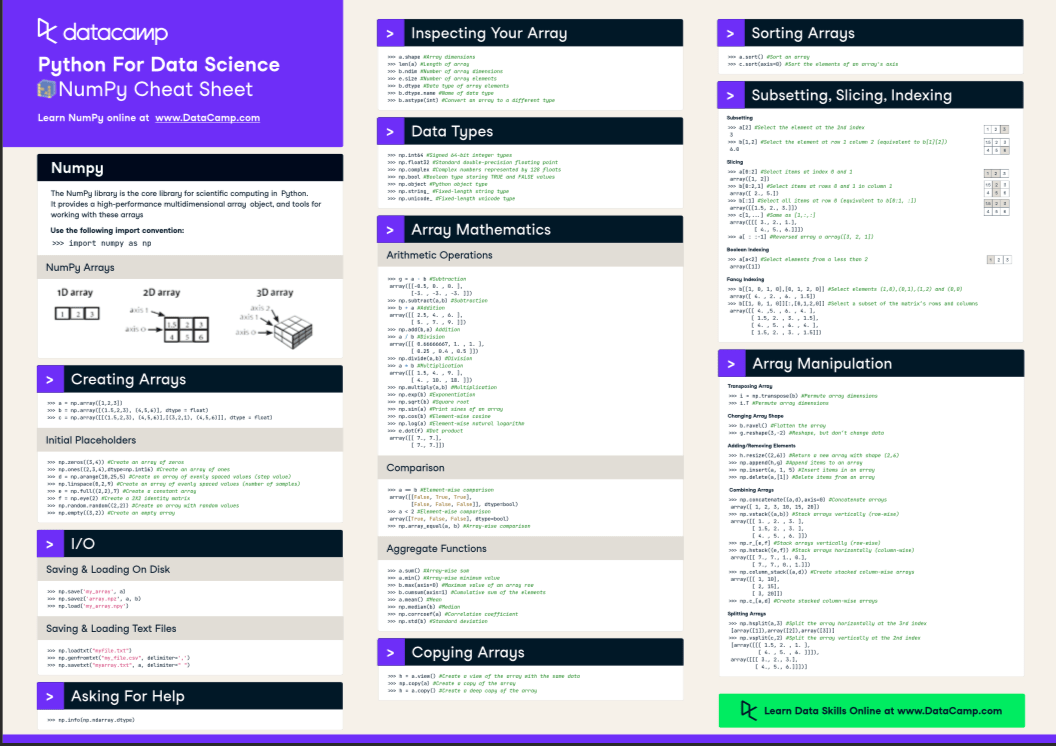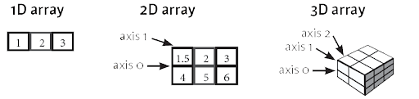# NumPy Cheat Sheet: Data Analysis in Python

This Python cheat sheet is a quick reference for NumPy beginners.

Given the fact that it's one of the fundamental packages for scientific computing, NumPy is one of the packages that you must be able to use and know if you want to do data science with Python. It offers a great alternative to Python lists, as NumPy arrays are more compact, allow faster access in reading and writing items, and are more convenient and more efficient overall.

In addition, it's (partly) the fundament of other important packages that are used for data manipulation and machine learning which you might already know, namely, Pandas, Scikit-Learn and SciPy:

• The Pandas data manipulation library builds on NumPy, but instead of the arrays, it makes use of two other fundamental data structures: Series and DataFrames,
• SciPy builds on Numpy to provide a large number of functions that operate on NumPy arrays, and
• The machine learning library Scikit-Learn builds not only on NumPy, but also on SciPy and Matplotlib.

You see, this Python library is a must-know: if you know how to work with it, you'll also gain a better understanding of the other Python data science tools that you'll undoubtedly be using.

It's a win-win situation, right?

Nevertheless, just like any other library, NumPy can come off as quite overwhelming at start; What are the very basics that you need to know in order to get started with this data analysis library?

This cheat sheet means to give you a good overview of the possibilities that this library has to offer.

Go and check it out for yourself!You'll see that this cheat sheet covers the basics of NumPy that you need to get started: it provides a brief explanation of what the Python library has to offer and what the array data structure looks like, and goes on to summarize topics such as array creation, I/O, array examination, array mathematics, copying and sorting arrays, selection of array elements and shape manipulation.

NumPy arrays are often preferred over Python lists, and you'll see that selecting elements from arrays is very similar to selecting elements from lists.

Do you want to know more? Check out DataCamp's Python list tutorial.

PS. Don't miss our other Python cheat cheets for data science that cover Scikit-LearnBokeh, Pandas and the Python basics.

NumPy

The NumPy library is the core library for scientific computing in Python. It provides a  high-performance multidimensional array object, and tools for working with these arrays.

Use the following improt convention:

``>>> import numpy as np``

Numpy Arrays## Creating Arrays

``````>>> a = np.array([1,2,3])
>>> b = np.array([(1.5,2,3), (4,5,6)], dtype = float)
>>> c = np.array([[(1.5,2,3), (4,5,6)],[(3,2,1), (4,5,6)]], dtype = float)``````

### Initial Placeholders

``````>>> np.zeros((3,4)) #Create an array of zeros
>>> np.ones((2,3,4),dtype=np.int16) #Create an array of ones
>>> d = np.arange(10,25,5)#Create an array of evenly spaced values (step value)
>>> np.linspace(0,2,9) #Create an array of evenlyspaced values (number of samples)
>>> e = np.full((2,2),7)#Create a constant array
>>> f = np.eye(2) #Create a 2X2 identity matrix
>>> np.random.random((2,2)) #Create an array with random values
>>> np.empty((3,2)) #Create an empty array
``````

## I/O

``````>>> np.save('my_array' , a)
>>> np.savez( 'array.npz', a, b)

``````>>> np.loadtxt("myfile.txt")
>>> np.genfromtxt("my_file.csv", delimiter= ',')
>>> np.savetxt( "myarray.txt", a, delimiter= " ")``````

``>>> np.info(np.ndarray.dtype)``

``````>>> a.shape #Array dimensions
>>> len(a)#Length of array
>>> b.ndim #Number of array dimensions
>>> e.size #Number of array elements
>>> b.dtype  #Data type of array elements
>>> b.dtype.name  #Name of data type
>>> b.astype(int). #Convert an array to a different type``````

## Data Types

``````>>> np.int64 #Signed 64-bit integer types
>>> np.float32. #Standard double-precision floating point
>>> np.complex. #Complex numbers represented by 128 floats
>>> np.bool  #Boolean type storing TRUE and FALSE values
>>> np.object #Python object type
>>> np.string_ #Fixed-length string type
>>> np.unicode_ #Fixed-length unicode type``````

## Array Mathematics

### Arithmetic Operations

``````>>> g = a - b. #Subtraction
array([[-0.5,0. ,0.], [-3. , -3. , -3. ]])
>>> np.subtract(a,b) #Subtraction
array([[ 2.5, 4. , 6.],[5. ,7. ,9. ]])
>>> a/b #Division
array([[0.66666667,1. ,1.],[0.25 ,0.4 ,0.5 ]])
>>> np.divide(a,b) #Division
>>> a * b #Multiplication
array([[1.5, 4. ,9.],[ 4. , 10. , 18. ]])
>>> np.multiply(a,b) #Multiplication
>>> np.exp(b) #Exponentiation
>>> np.sqrt(b) #Square root
>>> np.sin(a)  #Print sines of an array
>>> np.cos(b) #Elementwise cosine
>>> np.log(a)#Elementwise natural logarithm
>>> e.dot(f) #Dot product
array([[7.,7.],[7.,7.]])``````

### Comparison

``````>>> a == b #Elementwise comparison

array([[False , True, True],
[ False,False ,False ]], dtype=bool)
>>> a< 2 #Elementwise comparison
array([True, False, False], dtype=bool)
>>> np.array_equal(a, b) #Arraywise comparison``````

## Copying Arrays

``````>>>h = a.view()#Create a view of the array with the same data
>>> np.copy(a) #Create a copy of the array
>>>h = a.copy() #Create a deep copy of the array``````

## Sorting Arrays

``````>>> a.sort() #Sort an array
>>> c.sort(axis=0) #Sort the elements of an array's axis``````

## Subsetting, Slicing, Indexing

Subsetting

``````>>> a #Select the element at the 2nd index
3
>>> b[1,2] #Select the element at row 1 column 2(equivalent to b)
6.0``````

Slicing

``````>>> a[0:2]#Select items at index 0 and 1
array([1, 2])
>>> b[0:2,1] #Select items at rows 0 and 1 in column 1
array([ 2.,5.])
>>> b[:1]
#Select all items at row0(equivalent to b[0:1, :])
array([[1.5, 2., 3.]])
>>> c[1,...] #Same as[1,:,:]
array([[[ 3., 2.,1.],[ 4.,5., 6.]]])
>>> a[ : : -1] #Reversed array a array([3, 2, 1])``````

Boolean Indexing

``````>>> a[a<2] #Select elements from a less than 2
array()``````

Fancy Indexing

``````>>> b[[1,0,1, 0],[0,1, 2, 0]] #Select elements(1,0),(0,1),(1,2) and(0,0)
array([ 4. , 2. , 6. ,1.5])
>>> b[[1,0,1, 0]][:,[0,1,2,0]] #Select a subset of the matrix’s rows and columns
array([[ 4. ,5. , 6. , 4.],[1.5, 2. , 3. ,1.5],[ 4. ,5. , 6. , 4.],[1.5, 2. , 3. ,1.5]])

``````

## Array Manipulation

Transposing Array

``````>>> i = np.transpose(b) #Permute array dimensions
>>> i.T #Permute array dimensions``````

Changing Array Shape

``````>>> b.ravel() #Flatten the array
>>> g.reshape(3, -2) #Reshape, but don’t change data``````

``````>>>h.resize((2,6)) #Return a new arraywith shape(2,6)
>>> np.append(h,g) #Append items to an array
>>> np.insert(a,1,5)  #Insert items in an array
>>> np.delete(a,)  #Delete items from an array``````

Combining Arrays

``````>>> np.concatenate((a,d),axis=0) #Concatenate arrays
array([1, 2, 3, 10, 15, 20])
>>> np.vstack((a,b) #Stack arrays vertically(row wise)
array([[1. , 2. , 3.],[1.5, 2. , 3.],[ 4. ,5. , 6. ]])
>>> np.r_[e,f] #Stack arrays vertically(row wise)
>>> np.hstack((e,f)) #Stack arrays horizontally(column wise)
array([[7.,7.,1.,0.],[7.,7.,0.,1.]])
>>> np.column_stack((a,d)) #Create stacked column wise arrays
array([[1, 10],[ 2, 15],[ 3, 20]])
>>> np.c_[a,d] #Create stacked column wise arrays``````

Splitting Arrays

``````>>> np.hsplit(a,3) #Split the array horizontally at the 3rd index
[array(),array(),array()]
>>> np.vsplit(c,2) #Split the array vertically at the 2nd index
[array([[[ 1.5, 2. ,1.],[ 4. ,5. , 6. ]]]),
array([[[ 3., 2., 3.],[ 4.,5., 6.]]])]``````

Have this Cheat Sheet at your fingertips